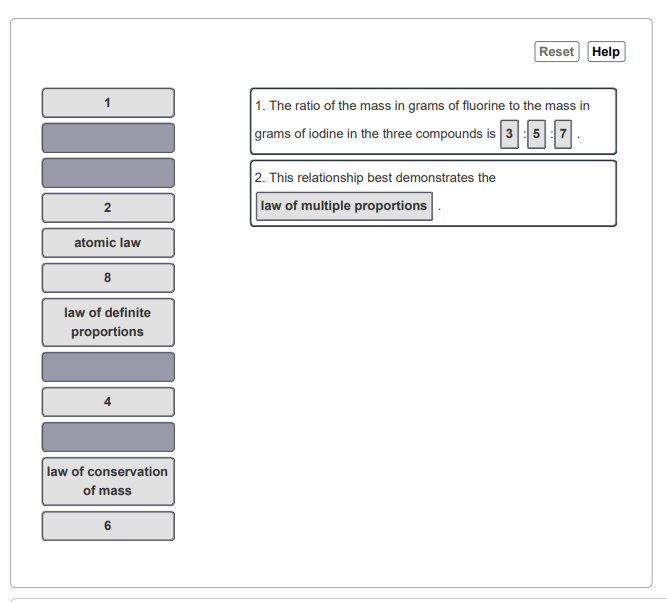# Problem: In a series of experiments, a chemist prepared three different compounds that contain only iodine and fluorine and determined the mass of each element in each compound:Compound Mass of iodine (g)  Mass of fluorine (g) 14.753.5627.643.4339.419.86(b) Analyze how the numbers in the previous parts support atomic theory.  Match the integers and words in the left column to the appropriate blanks in the sentences on the right. Make certain each sentence is complete before submitting your answer.

###### FREE Expert Solution

Use the formula:

• Cpd 1 = 0.749
• Cpd 2 = 0.449
• Cpd 3 = 1.05

86% (356 ratings)###### Problem Details
In a series of experiments, a chemist prepared three different compounds that contain only iodine and fluorine and determined the mass of each element in each compound:

 Compound Mass of iodine (g) Mass of fluorine (g) 1 4.75 3.56 2 7.64 3.43 3 9.41 9.86

(b) Analyze how the numbers in the previous parts support atomic theory.  Match the integers and words in the left column to the appropriate blanks in the sentences on the right. Make certain each sentence is complete before submitting your answer.Frequently Asked Questions

What scientific concept do you need to know in order to solve this problem?

Our tutors have indicated that to solve this problem you will need to apply the Law of Multiple Proportions concept. You can view video lessons to learn Law of Multiple Proportions. Or if you need more Law of Multiple Proportions practice, you can also practice Law of Multiple Proportions practice problems.

What professor is this problem relevant for?

Based on our data, we think this problem is relevant for Professor Sommer's class at MIAMIOH.